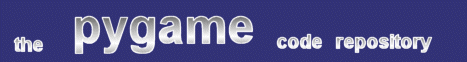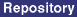### Title: LOS checking using line-segment intersection

Author: David Clark (silenus at telus.net)
Submission date: April 03, 2001

Description: Adapted from code by Mukesh Prasad in Graphics Gems II, this code checks to see if two objects can see one another on a 2d plane. Each object is assumed to have a .rect member, which was supplied from the pygame.Rect() function. Each object in the game that could possibly block line of sight must have its rect added to a list, which is supplied to the can_see() function. This code is the basis of the LOS-checking code in my game, Twitch.

pygame version required: Any
SDL version required: Any
Python version required: Any

Comments: The code in the line_seg_intersect function could probably be improved; there's a great deal of excess assignment going on.

```
# Constants for line-segment tests
DONT_INTERSECT = 0
COLINEAR = -1

def have_same_signs(a, b):
return ((a ^ b) >= 0)

def line_seg_intersect(line1point1, line1point2, line2point1, line2point2):
x1 = line1point1
y1 = line1point1
x2 = line1point2
y2 = line1point2
x3 = line2point1
y3 = line2point1
x4 = line2point2
y4 = line2point2

a1 = y2 - y1
b1 = x1 - x2
c1 = (x2 * y1) - (x1 * y2)

r3 = (a1 * x3) + (b1 * y3) + c1
r4 = (a1 * x4) + (b1 * y4) + c1

if ((r3 != 0) and (r4 != 0) and have_same_signs(r3, r4)):
return(DONT_INTERSECT)

a2 = y4 - y3
b2 = x3 - x4
c2 = x4 * y3 - x3 * y4

r1 = a2 * x1 + b2 * y1 + c2
r2 = a2 * x2 + b2 * y2 + c2

if ((r1 != 0) and (r2 != 0) and have_same_signs(r1, r2)):
return(DONT_INTERSECT)

denom = (a1 * b2) - (a2 * b1)
if denom == 0:
return(COLINEAR)
elif denom < 0:
offset = (-1 * denom / 2)
else:
offset = denom / 2

num = (b1 * c2) - (b2 * c1)
if num < 0:
x = (num - offset) / denom
else:
x = (num + offset) / denom

num = (a2 * c1) - (a1 * c2)
if num <0:
y = (num - offset) / denom
else:
y = (num - offset) / denom

return (x, y)

def can_see(source, target, blocking_rects_list):

"""
Performs a los check from the center of the source to the center of the target.
Makes the following assumtion:
1 - Both the source and target are objects that include a pygame.Rect() member object
called object.rect.

Returns 1 of line of sight is clear. Returns 0 if it is blocked.
"""

los_line_p1 = source.rect.center
los_line_p2 = target.rect.center

# check each candidate rect against this los line. If any of them
# intersect, the los is blocked.

for rect in blocking_rects_list:
block_p1 = rect.topleft
block_p2 = rect.bottomright
if line_seg_intersect(los_line_p1, los_line_p2, block_p1, block_p2):
return 0
block_p1 = rect.topright
block_p2 = rect.bottomleft
if line_seg_intersect(los_line_p1, los_line_p2, block_p1, block_p2):
return 0
return 1

```
 From: Anonymous Date: December 19, 2004 19:33 GMT this does not work!!

 Feedback